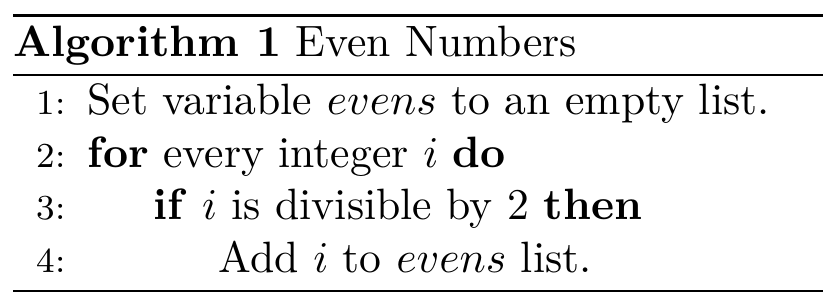# ~/Blog

## Brandon RozekPhD Student @ RPI studying Automated Reasoning in AI and Linux Enthusiast.

# Algorithms in LaTex

Published on

Updated on

There’s a great package in LaTex called algorithm to help format psuedo-code algorithms for scientific papers. Here’s a simple example of its usage:

\usepackage{algorithm}
\usepackage[noend]{algpseudocode}
\begin{algorithm}
\caption{Even Numbers}
\begin{algorithmic}
\State Set variable $evens$ to an empty list.
\For {every integer $i$}
\If {$i$ is divisible by $2$}
\State Add $i$ to $events$ list.
\EndIf
\EndFor
\end{algorithmic}
\end{algorithm}Have you published a response to this? :